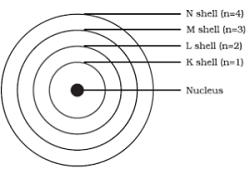### Structure of the Atoms - Solutions

CBSE class IX Science

NCERT Solutions
Chapter - 4
Structure of the Atom

(Page No. 47)

1. What are canal rays?

Ans. E. Goldstein discovered positively charged rays, those rays are called canal rays. These rays travel in a direction opposite to cathode rays. Canal rays led to the discovery of positively charged sub atomic particle known as proton.

2. If an atom contains one electron and one proton, will it carry any charge or not?

Ans. That atom will not contain any charge because one negative charge of single electron and one positive charge of single proton are neutralized by each other.

(Page No. 49)

1. On the basis of Thomson’s model of an atom, explain how the atom is neutral as a whole.

Ans. As per Thomson’s model of an atom, the number of electrons (negatively charged) are equal to the number of protons (positively charged) in an atom. Hence the +ve and –ve charges are neutralized by each other that makes atom neutral as a whole.

2. On the basis of Rutherford’s model of an atom, which subatomic particle is present in the nucleus of an atom?

Ans. The sub atomic particle proton is present in the nucleus of an atom according to Rutherford’s model of an atom.

3. Draw a sketch of Bohr’s model of an atom with three shells.

Ans.4. What do you think would be the observation if the α-particle scattering experiment is carried out using a foil of a metal other than gold?

Ans. No, the observations would have been same even if the α-particle scattering experiment is carried out using a foil of a metal other than gold.

5. Name the three sub-atomic particles of an atom.

Ans. Proton, Neutron and Electron.

6. Helium atom has an atomic mass of 4 u and two protons in its nucleus. How many neutrons does it have?

Ans. The number of neutrons present in a helium atom = atomic mass – no. of protons

= 4-2 = 2

(Page No. 50)

1. Write the distribution of electrons in carbon and sodium atoms.

Ans. Distribution of electrons in carbon atom: atomic number of carbon = 6 = 2,4

Distribution of electrons in sodium atom: atomic number of sodium = 11 = 2,8,1

2. If K and L shells of an atom are full, then what would be the total number of electrons in the atom?

Ans. If K and L shells of an atom are full, then the total number of electrons in the atom will be 10 because K shell can accommodate total 2 and L shell can accommodate maximum 8 electrons that makes a total of 10.

(Page No. 52)

1. How will you find the valency of chlorine, sulphur and magnesium?

Ans. The electrons present in the outermost shell of an atom are known as the valence electrons. Those electrons determine the valency of that atom.

The atomic number of chlorine is 17 = 2,8,7

so the number of valence electrons for chlorine is 7 and it needs 1 more electron to complete its octet (8). Therefore, its valency is one.

similarly, Sulphur = 16 = 2,8,6

so the number of valence electrons for sulphur is 6 and it needs 2 more electrons to complete its octet (8). Therefore, its valency is two.

similarly, for magnesium = 12 = 2,8,2

It becomes easier for magnesium to give away its two valence electrons than to acquire 6 more therefore its valency is two.

2. If number of electrons in an atom is 8 and number of protons is also 8, then

(i) what is the atomic number of the atom? and

(ii) what is the charge on the atom?

Ans. (i) atomic number = number of protons = number of electrons = 8

(ii)atom will be neutral (no charge) because number of protons(+) is equal to the number of electrons (-).

3. With the help of Table 4.1, find out the mass number of oxygen and sulphur atom.

Ans. mass number of oxygen = number of neutrons + number of protons = 8+8 =16

mass number of sulphur = number of neutrons + number of protons = 16 + 16 = 32

(Page No. 53)

1. For the symbol H,D and T tabulate three sub-atomic particles found in each of them.

Ans.

 Symbol Number of electrons Number of protons Number of neutrons H(Hydrogen) 1 1 0 D (Deuterium) 1 1 1 T (Tritium) 1 1 2

2. Write the electronic configuration of any one pair of isotopes and isobars.

Ans. Isotopes of carbon: 126C and 136C Electronic configuration is 2,4 because both the atoms have the same atomic number and the same number of electrons as 6.

Isobars:
4020Ca - 2,8,8,2 as atomic number is 20  and 4018Ar - 2,8,8 as atomic number is 18